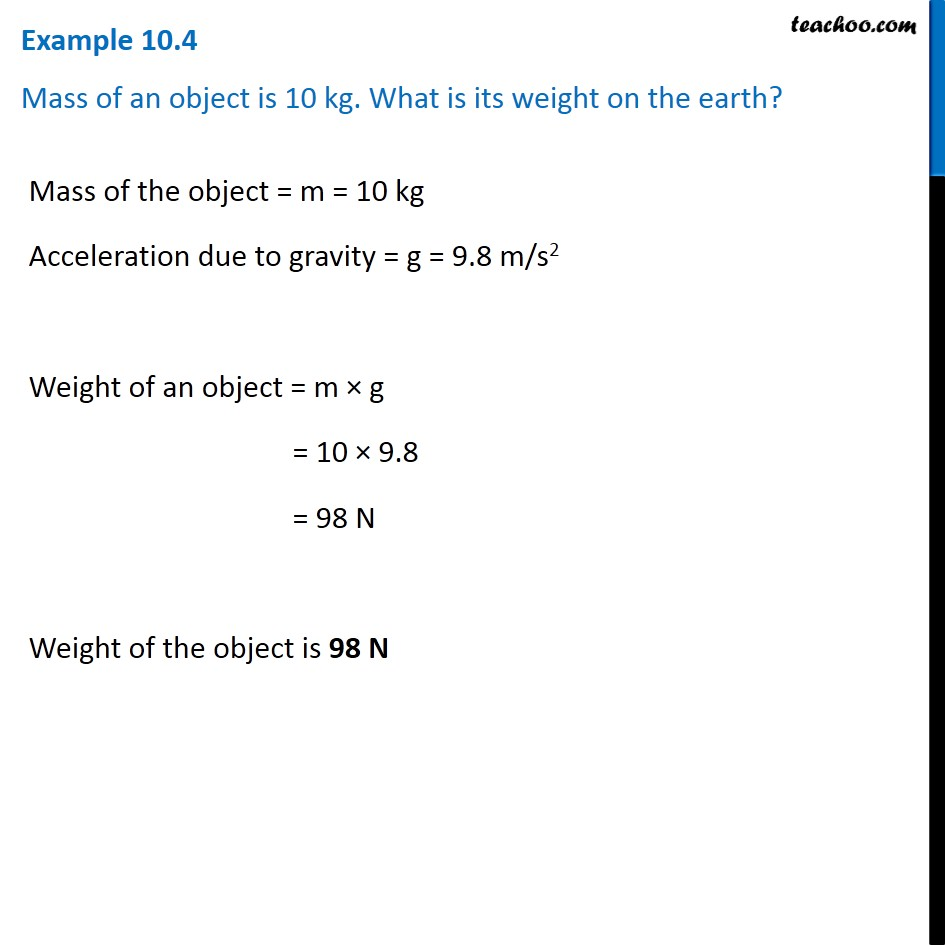Examples from NCERT Book

Class 9
Chapter 10 Class 9 - GravitationLearn in your speed, with individual attention - Teachoo Maths 1-on-1 Class

### Transcript

Example 10.4 Mass of an object is 10 kg. What is its weight on the earth? Mass of the object = m = 10 kg Acceleration due to gravity = g = 9.8 m/s2 Weight of an object = m × g = 10 × 9.8 = 98 N Weight of the object is 98 N